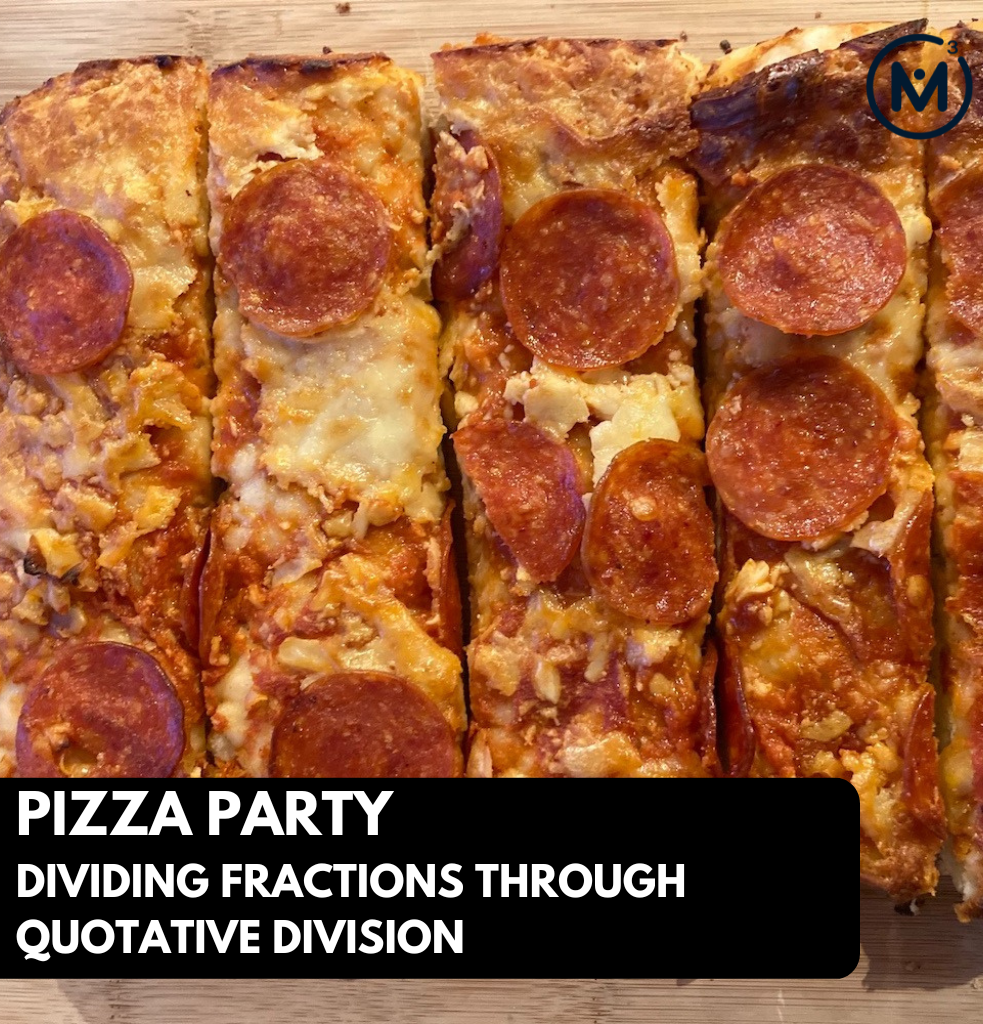## PIZZA PARTY [DAY 2]

### DIVIDING FRACTIONS THROUGH QUOTATIVE DIVISION

Explore quotative division of fractions conceptually using the bar model, double number line and ratio table.

## Intentionality & Unit Overview### Access each lesson from this unit using the navigation links below

Students will divide fractions through quotative division leveraging a context.

### Intentionality…

The purpose of the Day 2 activities is to reinforce key concepts from Day 1. Students will engage in a string of related problems through a math talk and will have an opportunity to complete independent purposeful practice. The math talk and purposeful practice serve to develop a deeper understanding of the following big ideas:

• There are two types of division;
• In quotative division, both the dividend and the divisor have the same unit (pizza and pizza).
• The divisor is a rate.
• The quotient can represent the number of parts or iterations of the rate.
• Quotative division can be accessed through skip counting, repeated addition, repeated subtraction or multiplication;
• When you scale both the dividend and the divisor by the same factor, the quotient will remain the same.

## Math Talk

Present each of the following division statements one at a time. Leverage yesterday’s context, pizzas and pizza/serving. You might consider using an area model to support student thinking.

#### 3 pizzas ÷ 1 $$\frac{1}{2}$$ pizzas/serving

Login/Join to access the entire Teacher Guide, downloadable slide decks and printable handouts for this lesson and all problem based units.

At the end of this string, students might be able to make a conjecture.

When you half both the number of pizzas, and the size of the serving, the number of servings stays the same. Is this always true? What if you double the number of pizzas and double the size of each serving? Is this the same for tripling? Allow students to explore this relationship and make a generalization about this behavior of division.

## While Students Are Practicing…

Login/Join to access the entire Teacher Guide, downloadable slide decks and printable handouts for this lesson and all problem based units.

## Purposeful Practice: Dividing Fractions

### Question #1:

Login/Join to access the entire Teacher Guide, downloadable slide decks and printable handouts for this lesson and all problem based units.

### Question #2:

Login/Join to access the entire Teacher Guide, downloadable slide decks and printable handouts for this lesson and all problem based units.

### Question #3:

Login/Join to access the entire Teacher Guide, downloadable slide decks and printable handouts for this lesson and all problem based units.

### Question #4:

Login/Join to access the entire Teacher Guide, downloadable slide decks and printable handouts for this lesson and all problem based units.

We suggest collecting this reflection as an additional opportunity to engage in the formative assessment process to inform next steps for individual students as well as how the whole class will proceed.

Login/Join to access the entire Teacher Guide, downloadable slide decks and printable handouts for this lesson and all problem based units.

## Educator Discussion Area

Login/Join to access the entire Teacher Guide, downloadable slide decks and printable handouts for this lesson and all problem based units.

## Explore Our 60+ Problem Based Units

This Make Math Moments Lesson was designed to spark curiosity for a multi-day unit of study with built in purposeful practice, number talks and extensions to elicit and emerge strategies and mathematical models.

Dig into our other units of study and view by concept continuum, grade or topic!